HOME Electronics Directory Articles/ Tutorials eBooks About Us FORUM Links Contact Us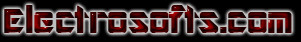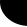Parallel Port Programming (Part 2): with Visual C++ Harsha Perla

Now, you are knowing the pins and registers of parallel port. You know how to access them in DOS. If you want to run your program in Windows 95 or 98, you are having access to the port in the similar way. You need to know how to use dialog boxes and windows materials with it. But your program should also run in Windows XP, NT or higher versions, then there is another issue. Higher versions of Windows do not allow to access the hardware directly for security reasons. But still, there are ways, I will explain later. First we will start programming which will work only in lover versions of Windows.

Direct Access:

If you want to program the port in VB, there is no direct access to the port. Still you can access the port using DLL files created with VC++. You can use the next method Access using inpout32.

If you are familiar with Visual C++, then create a dialog based application named ParallelPort and skip this section, go to adding controls.

Creating the application:

• Start Visual C++, Select File->New.

• In the tab 'Projects', Select "MFC AppWizard (exe)", give project name as "ParallelPort" and click OK.

• In the next window, select radio button "Dialog based" and click next leaving all other options default.

• Click Finish, then OK to get a window with two buttons and one sentence "TODO: Place Dialog controls here.", select and delete that sentence. Click to select the button "Cancel" and delete it.

• Right click on button labeled OK, select Properties from the drop down menu. Change the value of Caption to "&EXIT" from "OK".

• Resize the dialog box to get a window as shown below. If you run the application by clicking this icon: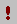, it should give 0 errors and 0 warnings, and you will get the following window.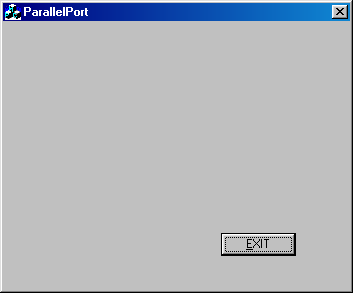figure(2.1)

 Now, you should see a tool bar as shown here, it is called Control toolbar. If not, select it from view menu->toolbars. Icon marked here with red color is the Check Box. If you click the check box icon and draw in the window, You will have the check box placed in the window. You need to place 17 such check boxes in the window. You can use copy-past to make your work easy. After that, Group then using 3 group boxes. Group box icon is there above the check box in the figure. After doing this much, your design should look like figure(2.3). So, re arrange your dialog components to look like that.  Again run the application and make sure that there is no error.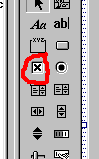figure(2.2)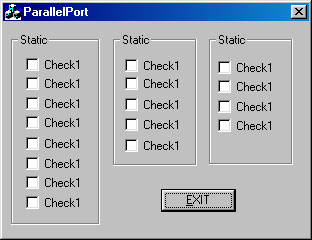Figure(2.3)

Next, right click on the Group Box labeled Static and go to properties. Change the captions to Data, Status and Control respectively. Right click on the first Check box Check 1, Change the caption to "Pin 2" and ID to IDC_Pin2. Similarly change the captions of check boxes in data group to Pin 2 to Pin 9; status port Pin 10, Pin 11, Pin 12, Pin 13 and Pin 15; Control Port Pin 1, Pin 14, Pin 16 and Pin 17. Change the ID's correspondingly(IDC_Pin2, IDC_Pin3...).

Window designing is over. Next part is coding. We have placed some controls in the dialog box. To get the values of these controls, we need to have variables associated with then. To do that, right click and select "ClassWizard" from drop down menu. Select tab "Member Variables". You will get a list of Control IDs. Select each IDs separately and click Add Variable. Type variable name as m_pin1. m_pin10, m_pin11... and retain Category Value and Variable type BOOL. Refer following figure.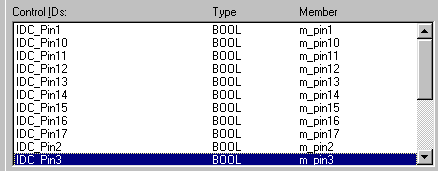Figure(2.4)

In the Workspace, Select ClassView tab, under ParallelPort classes, right click on CParallelPortDlg, click Add Member Function. Give function type as void and function name as UpdatePins(). It will take you to the new function created. Edit the code as follows.

 void CParallelPortDlg::UpdatePins() {      int reg;      reg=_inp(STATUS);      if((reg & 0x40)==0) m_pin10=0;     else m_pin10=1;      if((reg & 0x80)==0) m_pin11=0;     else m_pin11=1;      if((reg & 0x20)==0) m_pin12=0;     else m_pin12=1;          if((reg & 0x10)==0) m_pin13=0;     else m_pin13=1;      if((reg & 0x08)==0) m_pin15=0;     else m_pin15=1;      //////////      reg=_inp(DATA);           if((reg & 0x01)==0) m_pin2=0;     else m_pin2=1;      if((reg & 0x02)==0) m_pin3=0;     else m_pin3=1;          if((reg & 0x04)==0) m_pin4=0;     else m_pin4=1;      if((reg & 0x08)==0) m_pin5=0;     else m_pin5=1;      if((reg & 0x10)==0) m_pin6=0;     else m_pin6=1;      if((reg & 0x20)==0) m_pin7=0;     else m_pin7=2;      if((reg & 0x40)==0) m_pin8=0;     else m_pin8=1;      if((reg & 0x80)==0) m_pin9=0;     else m_pin9=1;      //////      reg = _inp(CONTROL);      if((reg & 0x01)==0) m_pin1=0;      else m_pin1=1;      if((reg & 0x02)==0) m_pin14=0;     else m_pin14=1;      if((reg & 0x04)==0) m_pin16=0;     else m_pin16=1;      if((reg & 0x08)==0) m_pin17=0;     else m_pin17=1;      UpdateData(FALSE); }

Now scroll to the top of the page and add these lines before class declarations.

 #define DATA 0x378 #define STATUS 0x379 #define CONTROL 0x37a

The function CParallelPortDlg::UpdatePins() is used to display values of all pins initially. Here, we have used _inp() function to get the values of registers associated with the ports. _inp(PORT) will return the data present in PORT. Depending on the status of the pins, we are making Check boxes checked or unchecked. When we change the value of member variable and call the function UpdateData(FALSE), the values in the member variables will be updated in the corresponding controls in the window. Similarly if you call UpdateData(TRUE), Values which are there in the corresponding controls will sit in the member variables. Here, The values from the variables should be updated in the window. So, UpdateWindow(FALSE). If you have read my first article, you will understand all other things done here.

To make run this code when the dialog is initialized, we need to call it. So, go to function OnInitdialog() in the file CParallelPortDlg. (In the class view tab of the workspace, under ParallelPort Classes, expand CParallelPortDlg, you will get the function name, double click it.) Add the following code to it. This code will call the function UpdatePins() and set a timer to scan the port pins. You can change the second parameter to change the frequency at which ports are needed to be scanned. I have used 200 milli seconds. _outp(CONTROL, 0xDF) will reset the control register bit 5 low so that data pins will act as output. _outp(PORT, DATA) sends the byte DATA to the address PORT.

 BOOL CParallelPortDlg::OnInitDialog() {     //App.Wiz generated code     // TODO: Add extra initialization here     SetTimer(1,200,NULL);     _outp(CONTROL, _inp(CONTROL) & 0xDF);     UpdatePins();     return TRUE; // return TRUE unless you set the focus to a control }

Next part is Updating the pin contents for each timer tics. For that, we need to handle the windows message WM_TIMER. Now since we have set the timer for 200 ms, for every 200 ms, Windows returns WM_TIMER message. To write a handler, write click on the CParallelPortDlg in the class view tab, select "Add Windows Message Handler...". In "New Windows messages/events", select WM_TIMER and click Add and Edit. It will take you to the newly created function CParallelPortDlg::OnTimer(UINT nIDEvent). Add the following code to it.

 void CParallelPortDlg::OnTimer(UINT nIDEvent) {     // TODO: Add your message handler code here and/or call default     int status_reg;     status_reg=_inp(STATUS);       if((status_reg & 0x40)==0) m_pin10=0;    else m_pin10=2;     if((status_reg & 0x80)==0) m_pin11=0;    else m_pin11=1;     if((status_reg & 0x20)==0) m_pin12=0;    else m_pin12=1;     if((status_reg & 0x10)==0) m_pin13=0;    else m_pin13=1;     if((status_reg & 0x08)==0) m_pin15=0;    else m_pin15=1;     UpdateData(FALSE);     CDialog::OnTimer(nIDEvent); }

Here, we have refreshed only input pins. Output pins have to be changed when user clicks on the check boxes. To find any change of value in check boxes, we can use BN_CLICKED message handler. But for all the check boxes we have to repeat the process. It is easy to use ON_COMMAND_RANGE. For that, scroll up to the position in the file ParallelPortDlg.cpp where you find BEGIN_MESSAGE_MAP(CParallelPortDlg, CDialog). (Do not confuse between CParallelPortDlg and CAboutDlg.)  Add the following code to it.

 BEGIN_MESSAGE_MAP(CParallelPortDlg, CDialog)      //{{AFX_MSG_MAP(CParallelPortDlg)      ON_WM_SYSCOMMAND()      ON_WM_PAINT()      ON_WM_QUERYDRAGICON()      ON_WM_TIMER()      //}}AFX_MSG_MAP      //Code added by me from here.      ON_COMMAND_RANGE(IDC_Pin2, IDC_Pin9, ChangePin)                ON_COMMAND(IDC_Pin14, ChangeControl)      ON_COMMAND(IDC_Pin16, ChangeControl)      ON_COMMAND(IDC_Pin17, ChangeControl)      ON_COMMAND(IDC_Pin1, ChangeControl)      //Code added by me till here END_MESSAGE_MAP()

Above code will call the function ChangePin() when buttons in the range IDC_Pin2 to IDC_Pin9 are changed and ChangeControl() when chack buttons with ID IDC_Pin14, IDC_Pin16, IDC_Pin17 or IDC_Pin1 are changed. Now we need those two functions. Add two new functions to CParllelPortDlg: void 'ChangePin()' and 'void ChangeControl()' using the method explained earlier. Write codes to them as follows:

 void CParallelPortDlg::ChangePin(int pin) { int data_register, new_register; UpdateData(TRUE); data_register=_inp( DATA ); new_register=0; if( m_pin2==TRUE ) new_register |= 0x01; if( m_pin3==TRUE ) new_register |= 0x02; if( m_pin4==TRUE ) new_register |= 0x04;   if( m_pin5==TRUE ) new_register |= 0x08; if( m_pin6==TRUE ) new_register |= 0x10; if( m_pin7==TRUE ) new_register |= 0x20; if( m_pin8==TRUE ) new_register |= 0x40; if( m_pin9==TRUE ) new_register |= 0x80; _outp(DATA, new_register); } void CParallelPortDlg::ChangeControl() { int control_register, new_register; UpdateData(TRUE); control_register = _inp( CONTROL ); new_register = control_register; if( m_pin1== 0 ) new_register &= 0xFE;     else new_register |= 0x01; if( m_pin14==0 ) new_register &= 0xFD;     else new_register |= 0x02; if( m_pin16==0 ) new_register &= 0xFB;     else new_register |= 0x04; if( m_pin17==0 ) new_register &= 0xF7;     else new_register |= 0x08; _outp(CONTROL, new_register); }

If everything is OK, you should get the following window when you run the program. To test the program, run this program without connecting anything to the port. Change some of the pins and close the window. If you run the program again you should get the values which was there before closing the window in the output pins.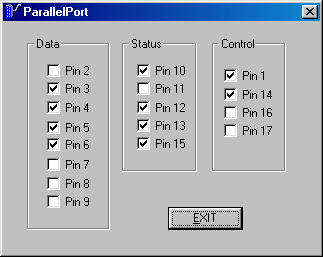You can always use this program to test the parallel port. Now, make a circuit connecting all the input pins to switches, all the output pins to LEDs with 2.2K or 10K resisters. If you press switch, corresponding pin value should change in the screen, If you change state of any output pin, corresponding LED should glow.

Every thing is ok. But as you know, this program will run only in win9x. If your program is needed to run in windows xp and higher versions, you need to write a kernel mode device driver. Do not worry if you are not up to that level. There are DLL files available freely for such drivers. You can use those files and call them from your program.

Access using inpout32

If you do not want to use driver and test the above program in Windows XP as it is, use my post in the ElectroSofts Forum

InpOut32 is a DLL file which can send a data to parallel port and which can return the data in the parallel port. You can download this file with source code for free from http://logix4u.net. You can use this file in any  windows programming language like Visual basic, C#, C++ etc. If you know how to use DLL files, download the file from http://logix4u.net and use Inp32() and Out32() functions instead of _inp() and _outp().

To know how to use DLL file in VC++, let us now convert our previous project to XP enabled program.

• Add these two lines in the file ParallelPortDlg.cpp after pre processor directives.

• Where ever _inp() comes, change them to Inp32() and where ever outp() comes, change them to Out32().

• Copy DLL file inpout32.dll and lib file inpout32.lib got by compiling the source code available at logix4u.net to the project folder.

• From project menu, select settings, go to tab link, in object/ library modules write inpout32.lib

• Now your program should run without any errors.

If you have any comment, feedback and suggestions, please send an e-mail to harsha@electrosofts.com or use this feedback form

To discuss the Interfacing related issues, use Electrosofts Forum